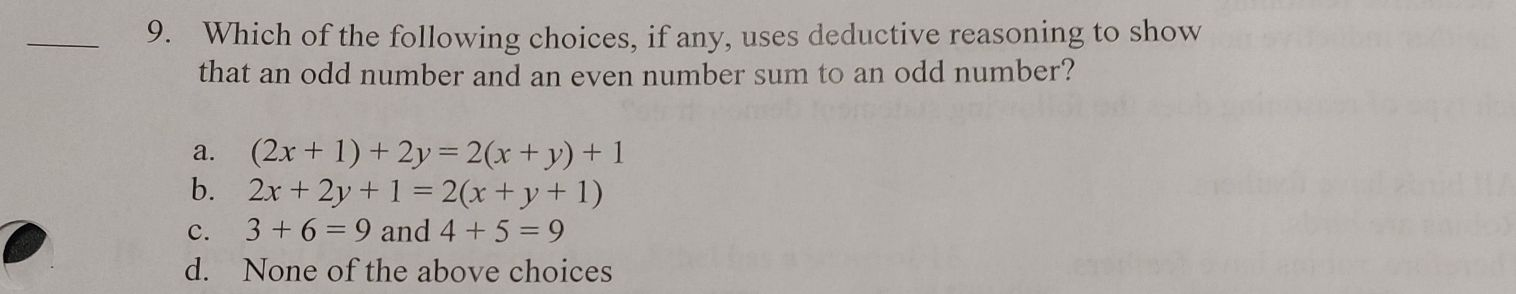### ¿Todavía tienes preguntas de matemáticas?

Pregunte a nuestros tutores expertos
Algebra
Pregunta9. Which of the following choices, if any, uses deductive reasoning to show that an odd number and an even number sum to an odd number?

a. $$( 2 x + 1 ) + 2 y = 2 ( x + y ) + 1$$

b. $$2 x + 2 y + 1 = 2 ( x + y + 1 )$$

c. $$3 + 6 = 9$$ and $$4 + 5 = 9$$

d. None of the above choices